GeeksforGeeks App
Open AppBrowser
Continue

# Using Timedelta and Period to create DateTime based indexes in Pandas

Real life data often consists of date and time-based records. From weather data to measuring some other metrics in big organizations they often rely on associating the observed data with some timestamp for evaluating the performance over time.

We have already discussed how to manipulate date and time in pandas, now let’s see the concepts of Timestamp, Periods and indexes created using these.

Using Timestamps :
Timestamp is the pandas equivalent of Python’s Datetime and is interchangeable with it in most cases. It denotes a specific point in time. Let’s see how to create Timestamp.

 `# importing pandas as pd``import` `pandas as pd`` ` `# Creating the timestamp``ts ``=` `pd.Timestamp(``'02-06-2018'``)`` ` `# Print the timestamp``print``(ts)`

Output :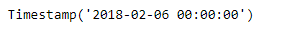Timestamps alone is not very useful, until we create an index out of it. The index that we create using the Timestamps are of `DatetimeIndex` type.

 `# importing pandas as pd``import` `pandas as pd`` ` `# Let's create a dataframe``df ``=` `pd.DataFrame({``'City'``:[``'Lisbon'``, ``'Parague'``, ``'Macao'``, ``'Venice'``],``                    ``'Event'``:[``'Music'``, ``'Poetry'``, ``'Theatre'``, ``'Comedy'``],``                    ``'Cost'``:[``10000``, ``5000``, ``15000``, ``2000``]})`` ` ` ` `# Let's create an index using Timestamps``index_ ``=` `[pd.Timestamp(``'01-06-2018'``), pd.Timestamp(``'04-06-2018'``),``          ``pd.Timestamp(``'07-06-2018'``), pd.Timestamp(``'10-06-2018'``)]`` ` `# Let's set the index of the dataframe``df.index ``=` `index_`` ` `# Let's visualize the dataframe``print``(df)`

Output :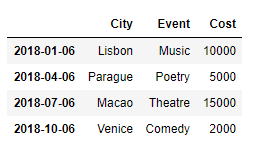Now we will see the type of dataframe index which is made up of individual timestamps.

 `# Check the type``print``(``type``(df.index))`

Output :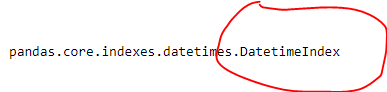As we can clearly see in the output, the type of the index of our dataframe is ‘DatetimeIndex’.

Using Periods : Unlike Timestamp which represents a point in time, Periods represents a period of time. It could be a month, day, year, hour etc.. Let’s see how to create Periods in Pandas.

 `# importing pandas as pd``import` `pandas as pd`` ` `# Let's create the Period``# We have created a period``# of a month``pr ``=` `pd.Period(``'06-2018'``)`` ` `# Let's print the period``print``(pr)`

Output :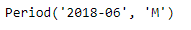The ‘M’ in the output represents month.

Period objects alone is not very useful until it is used as an Index in a Dataframe or a Series. An index made up of Periods are called `PeriodIndex`.

 `# importing pandas as pd``import` `pandas as pd`` ` `# Let's create a dataframe``df ``=` `pd.DataFrame({``'City'``:[``'Lisbon'``, ``'Parague'``, ``'Macao'``, ``'Venice'``],``                    ``'Event'``:[``'Music'``, ``'Poetry'``, ``'Theatre'``, ``'Comedy'``],``                    ``'Cost'``:[``10000``, ``5000``, ``15000``, ``2000``]})`` ` ` ` `# Let's create an index using Periods``index_ ``=` `[pd.Period(``'02-2018'``), pd.Period(``'04-2018'``),``          ``pd.Period(``'06-2018'``), pd.Period(``'10-2018'``)]`` ` `# Let's set the index of the dataframe``df.index ``=` `index_`` ` `# Let's visualize the dataframe``print``(df)`

Output :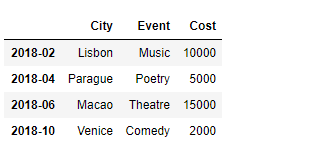Now we will see the type of our dataframe index which is made up of individual Periods.

 `# Check the type``print``(``type``(df.index))`

Output :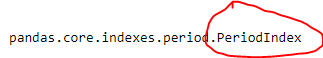As we can see in the output, the index created using periods are called PeriodIndex.

My Personal Notes arrow_drop_up